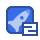# 算法导论中文版答案2收藏

NSERTION-SORT(A) for j+2 to length[A] do key←A的 //Insert AD] into the sorted sequence A[1. j-1 l·」 while i>o and askey doA[+1]·A[的 i←-j-1 A[+1]←key Algorithm 1 LINEAR-SEARCH(A, V Input: A=a1, a2,,. an) and a value v Output: An index i such that v= Ali or nil ifv2A 讠←1 Tondo if Ali]-y then return L d if return nil As a loop invariant wc say that none of the elcmcnts at index All,...,i 1] arc cqual to v. Clcarly, all properties arc fullfllcd by this loop invariant A、B各存放了一个二进制n位整数的 BINARY-ADD(A,B,C) 各位数值,现在通过二进制的加法对1fag-0 这两个数进行计算,结果以二进制形2forj-1ton 式把各位上的数值存放在数组C中 3 do 4key←A]+B+fag key存储临时计算结果,fag为进位 5 C0+ key mod 2 标志符,按位相加,8、9行不能少 if key >1 fad←1 g 8 if flag=1 C[n+们 n3/100-10n2-100n+3=O(n3) Assume that FIND-MIN(A, r, s returns the index of the smallest element in A between indices r and s. Clearly, this can be implemented in O(s -r)time ifr>s Algorithm 2 SELECTION SORT(A) Input: A=a1, a2,...anI Output: sorted A Or L TIND-MIN(A, 1, n) Ai+÷Ai end for As a loop invariant we choose that Al,...,i-I] are sorted and all other elements are greater than these. We only need to iterate to m-1 since according to the invariant the nth element will then the largest The n calls of FIND-MIN gives the following bound on the timc complexity This holds for both the best and worst -case running time Given that each element is equally likely to be the one searched for and the element searched foris present in the array, a linear search will on the average have to search through half th e elements Tais is because half the time the wanted element will be in the first half and half the time it will be in the second half. Doth the worst-case and average-case of LINEAR-SEARCH is O(n) Modify the algorithm so it tests whether the input satisfies some special-case con dition and, if It does, output a pre-computed answer. The best-case running time Is generally not a good measure of an algorithm 如下所示: 3,9,26,38,41,49,52,57 3,26,41,52 9,38,49,57 26,52 38,57 9,49 52 构建左半部分和右半部分的辅助数组 由题意得: 根据数学归纳法: 给出基本条件( base case) 当n=2时,T(2)=2l2=2。符合条件。 给出假设: 当n2时,T(2)=212成立。 那么, 当n=2时,T(21)=2T(21/2)+2 2T(22)+2+ 2(2g2t)+2 2+1g21 所以得证! Since it takes O(n )time in the worst case to insert ALn] into the sorted array All.n-l, we get the recurrence e(1) ifn=1 (n)= T(n-1)+0(n)if n The solution to this recurrence is T(n)=9(r) 给出一个递归算法.显然最坏情况的运行时间为(gn) BINARY-SEARCH(A, v, p, r) ifp2 r and y≠A[p]then return nil end if [ n/2」 j←Al(r-p)/2】 if v=al] then return e se if v<All then return BINARY-SEARCH(A; v,p; j) ese return BINARY-SEARCH(A; v;i;r) en 将插入排序的顺序查找改为二分查找的算法: I NSERTION-SORT(A) 9forj←2 to length 10 do key - a 11 △inse ul into the sorted sequence a[1.j-1] high←j-1 14 while low s high 15 mid = low+ high)/ 2 16 If key==A[mid then break 18 ikey≤A[md]then 19 high←mid-1 if key>Amid] then 21 Ow id +1 id toj1 A[i+1]←a[i Am]←key 在最坏情況下,二分查找的时间复杂度是rlgn,但插入时数组移动的时间复杂度仍是n。 故总体运行时间不能改善为ongn)。(但若排序中采用链表的数据结构,则可改善。) Give a O(nlgn] time algoriThm for deternining if there exist two elements in an set S whose sum eXac some value x Algorithm 4 CHECKSUMS(A, X Input: An array A and a value x. Output: A boolean valuc indicating if there is two clements in A whose sum is x A∈SORI(A ength] fori← to n do if Ali>0 and BINARY-SEARCh(A, Ali-x, 1, n) then return trle end if end for return false Clearly, this algorithm dces the job. (It is assumed that nil cannot be true in the if-statement. 第章 因为f)和g都是渐近非负的函数,所以根据定义有:存在NN2使得:当nN时f(n≥20, 同时,当n>N2时,g(n)>0.所以,我们取N。=max(NN此时,当n>N时,同时有f(n)>0,s(n)>0. 下面我们取C1=1/2,C2=1,根据f(n),g()的非负性保证,当n№时,有: f(n)+g(n) ≤maxf(),g(n)}≤f(n)+g(n) 所以,得证! To show that (n +a)=e(n), we want to find constants cl, C2, 7o>0 such that 0≤an≤(m+a)≤c2 no for all n≥ Note that 7+a≤n+|a when a|≤n and a≥n-|a when al≤n Thus, when n≥2|a 0≤=≤n+a≤2n Since b>0, the inequality still holds when all parts are raised to the power b 0≤(n)≤(n+a)≤(2n), 2)n≤(m+a)≤2n2 Thus, C1=(1/2), C2=26, and no=2 lal satisfy the definition Let the running time be T(n). T(n)20(n)means that T(n)> f(n)for some function f(n) in the set O(n). This statement holds for any running time T(n) since the function g(n)=0 for all n is in O(n), and running times are always nonnegative Thus the statement tells us nothing about the running time 2+1=0(2)因为2+1=2x2≤2x2,所以成立 2=0(2)不成立。用反证法:如果有2=02成立,根据定义需要有:2n≤c2,由此需 要: slac.矛盾!对于任意大的n是不可能成立的,因为c是一个常数。 首先证明充分性:即由f(n)=e(g(m)推出f(n)}=0(g(n)且f(m=Q(g(nj) 根据定义:有存在NaCo,使得:当n>N时有:Cg(m)≤f(m)≤C1g(m)在根据O,和Q 定义有f(n)=O(g(n)且f(n)=Q(g(n) 在证明必要性:即由fn}=O(g(n)且fn=Q(g(m)推出fn)=eg(m) 根据O,和Ω定义有:存在№,C1使得:当n>N1时有f(n)≤C1gn),同样地,存在N2,Co 使得:当n>N2时有cog(n)≤f(n),此时,我取N=max(N1,N2.则有如下事实成立:当n>No 时有:Cg(m≤f(n)≤C1g(m),即f(m)=egm)所以,得证! 由定义可易证,回记号渐近地给出—个函数的上界和下界,0记号给出一个函数的渐近上界 而g给出渐近下界。 Solution: Let fCo((n))n alg(n)). That means f=o(g(n)=w(g n). By the definition of o, we have lim(f(n)/g(2)=0 But the detinition ofo we have lim(f(n)/g(n))=co Becauise of0≠∞, we have a obvious contradiction Q(g(n,m))=f(n, m). there exist positive constants c, no, and mo such that0≤cg(n,m)≤f(n,m) for all n> no and m≥mo} o(g(n,m))=If(n, m). there exist positive constants cl, C2, no, and o such that0≤c8(n,m)≤f(m,m)≤C28(n,m) for all n≥ no and n1≥mo} 依题意可知,若n,≤n2 则有fm2)≤fn2),gn2)≤g(n2)f(m1)≤f(n2),g(m1)≤gn2 [f(n2)+g(m2)-[(m2)+g(m2)] f(n,)-f(n,)+g(n 故￡(n)+g(n)是单调递增的,后两个类似可得证。 logh a· logan= logh n· logh a commutativity of logh n 10gb/2 a (log x )y=log x (both sides) logh n_ logb c ogx. y(both sides) c>0,32>0,使得对所有的n≥,有0≤c2<n1,故|=w(2 如>033>0,使得对所有的nn有0≤n|≤cx3,故=O(12) 设「1gn1=m 则有m!=√2xmmc2m> 2 e (me)=e(nmt)>nlmm+ >n如an Solon! is not polynomially bounded Iglgn=m, m!<m<(2")=2m<2 andolan2m1-1,n≥22 glg|<2”≤ no So1glgn! is polynomially bounded. 后者大 数学归纳法易证 用数学归纳法证明

...展开详情

• 10
资源
• 2
粉丝
•等级算法导论中文版答案 50积分/C币 立即下载
1/6350积分/C币 立即下载